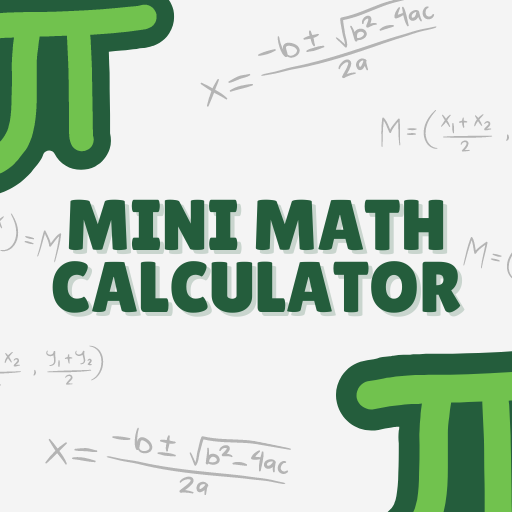## Mini Math Calculator

### by M Digital

Free
315
Categories Tags
###### Description

Mini Math Calculator is a specialized calculator designed specifically for solving math problems. It can perform basic arithmetic operations such as addition, subtraction, multiplication, and division, as well as more complex mathematical functions such as exponentiation, logarithms, and trigonometry.

https://minimilitiamods.net/
The calculator is user-friendly and provides quick and accurate results, making it a useful tool for students, professionals, and anyone who needs to perform mathematical calculations. With Mini Math Calculator, users can easily solve math problems and save time and effort.

• AOTD

2348
• AOTD

1119
• AOTD

279